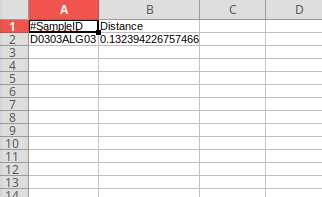# q2 - longitudinal first-distances only computes one distance

Hello,

I am a new user of Qiime2. I am trying to do some longitudinal anslysis using longitudinal first-distances (to calculate/plot the change in beta diverisity of samples over time). However, when I run the command, my output only contains one distance:This is my code:
qiime longitudinal first-distances
--i-distance-matrix beta_bray2.qza
--p-state-column Time
--p-individual-id-column Donation
--o-first-distances bray_distance_bray.qza

Here are my input files:
meta_long2.tsv (557 Bytes)
beta_bray2.qza (9.1 KB)

Any help will be greatly appreciatedThank you!
Carmen

1 Like

Hi @carmennns2 ,
Did you solve this? I see this topic where it looks like you have already computed the first distances.

It looks like the issue is that you collected samples at uneven intervals, i.e., some are at time 1, 6, 7, 11, or 12. `first-distances` assumes that samples are collected at even intervals (as otherwise the differences may be misleading), and determines the interval from the first sample that it encounters in the list.

The solution in this case would be to encode "order" as the state variable instead of time. I.e., it seems that you want to compare the baseline sample (time 1) to the second collection, vs. the third. So make a new metadata column with "order" as 1, 2, or 3. Then you are assessing the distance between sample collections based on the order that they were taken in instead of time (which is not consistent between donors so will not work in this case).

Good luck!